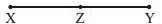# A and B walk from X to Y, a distance of 27 km at 5 km/hr and 7 km/hr, respectively, B reaches Y and immediately turns back meeting A at Z. What is the distance from X to Z? - Mathematics

MCQ

A and B walk from X to Y, a distance of 27 km at 5 km/hr and 7 km/hr, respectively, B reaches Y and immediately turns back meeting A at Z. What is the distance from X to Z?

• 25 km

• 22.5 km

• 24 km

• 20 km

#### Solution

22.5 km

Explanation:XY = 27

XZ =?

Speed of A = 5 km/hr, speed of B = 7 km/hr

Let in time t hr B cover XY and return to Z and A cover XZ

ATQ

5t + 7t = 54

12t = 54

"t"=54/12=9/2

xz=5xx9/2= 22.5 km

Concept: Time, Distance and Speed(Entrance Exam)
Is there an error in this question or solution?